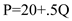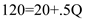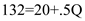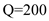# Quiz 35: Financial Economics

Business

In the short run, we will stay on the same aggregate supply curve. Thus, if the price level goes from 120 to 130 in the economy, we see that this will cause real output to shift from \$870 to \$890, since at a price level of 130 and staying on AS2 , the real output will be \$890. Thus, real output increases by \$20. In the long run, the economy will eventually shift back to the full employment level of output, which is given to be \$870. Therefore, in the long run, output will decrease by \$20 back to the \$870 potential output level. If the price level fell from 120 to 110, in the short run, the economy will stay on the same aggregate supply curve, AS2. Therefore, we see that on AS2 , a price level of 110 corresponds to a real output of \$850. Thus, real output falls from \$870 to \$850 in the short run, when the price level falls from 120 to 110. This is a fall of \$20. In the long run, the economy will eventually move back to the full employment level of output, which is given to be \$870. Therefore, in the long run, output will increase again by \$20 to go back to the \$870 potential output level. With every price level, the long run real output will be the economy's potential level of output, which is given to be \$870. Thus, the long run level of output at each of the three price levels is \$870.

In macroeconomics, the short run is a period in which nominal wages do not respond to changes in the price level. The long run is a period in which nominal wages fully responds to changes in the price level. In other words in short run input prices are inflexible or even perfectly inflexible whereas in the long run the input prices are fully flexible. The distinction is important because the differences in the assumption about the flexibility of input prices lead to large differences in the shape and position of the aggregate supply curve.

We are given that the equation for a short run AS curve is. If the price level, or P , iS120, then we have:. To find Q , we simply subtract both sides by 20 and divide both sides by.5, and we get:We can solve for how much Q would be if P went up to 132 by substituting 132 for P :. To find Q , we simply subtract both sides by 20 and divide both sides by.5, and we get:Thus, we see that in the short run, Q will increase by 24 (from 200 to 224). In the long run, Q will revert back to the full-employment level of output. We are given that the Q in the first partis the full-employment level of output. Therefore, Q will fall back to the full employment output of \$200 in the long run. In other words, Q will increase in the long run by -\$24.

There is no answer for this question